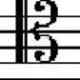#### [SOLVED] How does the Hubble parameter change with the age of the universe?

How does the Hubble parameter change with the age of the universe?

This question was posted recently, and I had almost finished writing an answer when the question was deleted. Since it's a shame to waste the effort here's the answer anyway.

Maybe this can be one of the canonical answers suggested by Danu.#### @Rob Jeffries 2017-05-15 12:09:39

A shorter version of John's answer that just focuses on what happens to the Hubble parameter in the future.

The solution to the Friedmann equation in a flat universe is $$H^2 = \frac{8\pi G}{3}\rho + \frac{\Lambda c^2}{3},$$ where $\rho$ is the matter density (including dark matter) and $\Lambda$ is the cosmological constant.

As the universe expands, $\rho$ of course decreases as $a(t)^{-3}$, but $\Lambda$ remains constant. Thus the first term on the RHS becomes unimportant if indeed $\Lambda$ is a cosmological constant.

Thus the Hubble parameter actually decreases from its current value $H_0$ and asymptotically tends towards $H = \sqrt{\Lambda c^2/3}$ as time tends towards infinity.

A more convenient way to write the above is to express all densities in terms of the critical density. In which case $$H^2 = H_0^2 (\Omega_m a^{-3} + \Omega_{\Lambda}),$$ where $H_0$ is the present day Hubble parameter, $\Omega_m$ is the present day ratio of matter density to the critical density, which evolves as the inverse cube of the scale factor $a$, and $\Omega_{\Lambda}$ is the (assumed) constant energy density of the vacuum expressed as a ratio with critical density.

Thus as $a$ gets big, then the Hubble parameter tends towards $H_0 \sqrt{\Omega_{\Lambda}} \sim H_0 \sqrt{2/3}$.#### @John Rennie 2014-09-18 08:53:04

To calculate the Hubble constant we need the a scale factor, $a(t)$. This is a measure of how much the universe has expanded. We take the scale factor to be unity at the current moment, so if $a = 2$ that means the universe has expanded twice as much as it has right now. Likewise $a = 0.5$ means the universe had expanded only half as much as it has right now. The Hubble constant is calculated from the scale factor using (see Pulsar's answer here for details):

$$H(a) = H_0\sqrt{\Omega_{R,0}\,a^{-4} + \Omega_{M,0}\,a^{-3} + \Omega_{K,0}\,a^{-2} + \Omega_{\Lambda,0}} \tag{1}$$

Calculating how $a$ varies with time is done using the equation (see Pulsar's answer again):

$$t(a) = \frac{1}{H_0}\int_0^a \frac{a'\,\text{d}a'}{\sqrt{\Omega_{R,0} + \Omega_{M,0}\,a' + \Omega_{K,0}\,a'^2 + \Omega_{\Lambda,0}\,a'^4}} \tag{2}$$

Doing the calculation isn't that hard. There is a Google spreadsheet with the calculation here. The values for the various parameters are taken from the Planck data:

\begin{align} H_0 &= 67.3\;\text{km}\,\text{s}^{-1}\text{Mpc}^{-1},\\ \Omega_{R,0} &= 9.24\times 10^{-5},\\ \Omega_{M,0} &= 0.315,\\ \Omega_{\Lambda,0} &= 0.685,\\ \Omega_{K,0} &= 0 \end{align}

And the results look like:The units of time are the current Hubble time, $1/H_0 \approx 14.5$ billion years, so $1$ on the time axis corresponds to $14.5$ billion years. Note that the line does not go through the point $(1,1)$ because the current Hubble time is greater than the age of the universe, $13.798$ billion years. This is because the expansion of the universe has been slowing with time since the Big Bang.

At early times we expect the scale factor to be dominated by matter, and this gives a $t^{2/3}$ dependence. At late times we expect the scale factor to be dominated by dark energy and this gives an exponential dependence on $t$. The graph shows this nicely, with the changeover being somewhere around half a Hubble time.

As a side issue, it's a pain not having an analytic formula for $a(t)$ so I fitted the following function to get a reasonably accurate approximate formula:

$$a(t) \approx c_1 t^{2/3} + c_2 \left( e^{t/c_3} - 1\right)$$

The best fit values for the coefficients were:

\begin{align} c_1 &= 0.822 \\ c_2 &= 0.0623 \\ c_3 &= 0.645 \end{align}

And the fit looks like:Not a bad fit, though be cautious about extrapolating beyond $t = 2/H_0$.

And finally we can calculate the Hubble constant using equation (1). Note the logarithmic scale - the Hubble constant goes to infinity as $t \rightarrow$ zero.:And the Hubble time is the just $1/H_0$:The graphs show that the Hubble constant is most definitely not constant, though it does tend to a constant value at late time. This is because the late time expansion is dominated by dark energy and the expansion becomes exponential with time. The exponential increase means there is a constant doubling time (the opposite of exponential decay where there is a constant half-life) and the doubling time is just the Hubble time. So the Hubble time tends to a constant.

I'll leave the post here. If anyone wants details of how equations (1) and (2) are obtained comment and I can add another answer with the gory details.#### @Danu 2014-09-18 09:00:26

I'd love to see the nitty gritty details ;)#### @Carl Witthoft 2014-09-18 11:29:44

Somehow my ancient twisted brain came up with "Double Hubble" and wants some old-fashioned chewing gum :-)#### @Jim 2014-09-18 14:38:37

Mmmmmm.... Double Hubble. The bubbles you blow with that chewing gum expand so fast...#### @John Rennie 2014-09-18 14:42:34

@Jim: no, no, no! That's extrinsic curvature!! :-)#### @Jim 2014-09-18 15:44:42

@JohnRennie But the interior of the bubble would have increasing space with intrinsic curvature#### @Emilio Pisanty 2015-12-22 22:47:07

Shameless advertising on meta does seem to work. Good Q&A pair!